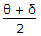# Civil Engineering - Advanced Surveying

### Exercise :: Advanced Surveying - Section 1

31.

The equation which is obtained by multiplying each equation by the coefficient of its un-knowns and by adding the equations thus formed, is known as

 A. observation equation B. conditional equation C. normal equation D. none of these.

Explanation:

No answer description available for this question. Let us discuss.

32.

Accidental errors

 A. do not follow any definite mathematical law B. cannot be removed by applying corrections to the observed values C. are generally small D. are also known as compensating errors E. all the above.

Explanation:

No answer description available for this question. Let us discuss.

33.

If θ and δ be the latitude of a place and declination of a star respectively, the upper culmination of the star will be north of zenith if its zenith distance, is

 A. δ - θ B. θ - δ C. θ + δ D..

Explanation:

No answer description available for this question. Let us discuss.

34.

In a tropical year, the number of sidereal days are

 A. one less than mean solar days B. one more than mean solar days C. equal to mean solar days D. none of these.

Explanation:

No answer description available for this question. Let us discuss.

35.

The position of a heavenly body on the celestial sphere can be completely specified by

 A. its altitude and azimuth B. its declination and hour angle C. its declination and right ascension D. all the above.